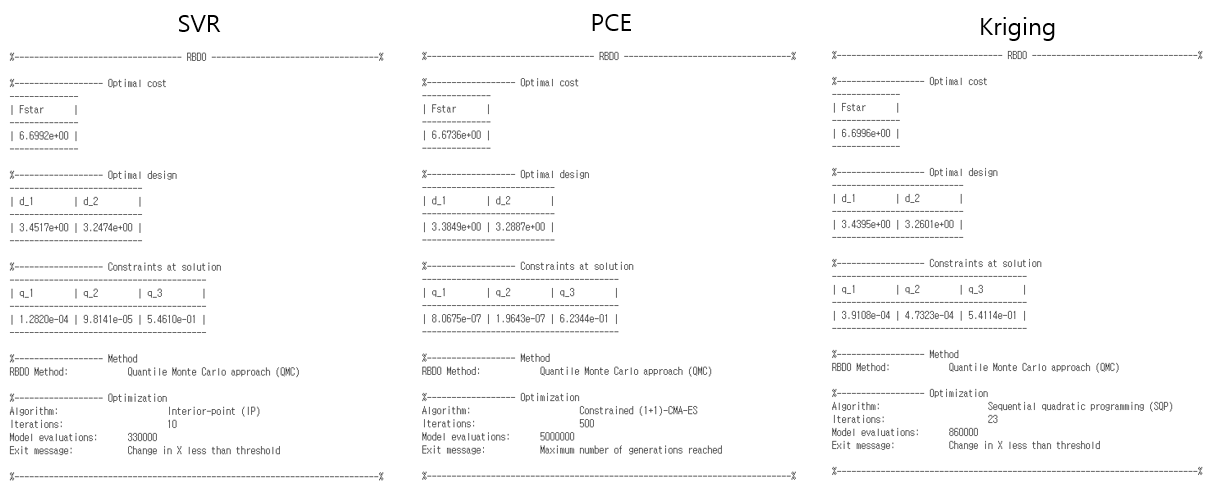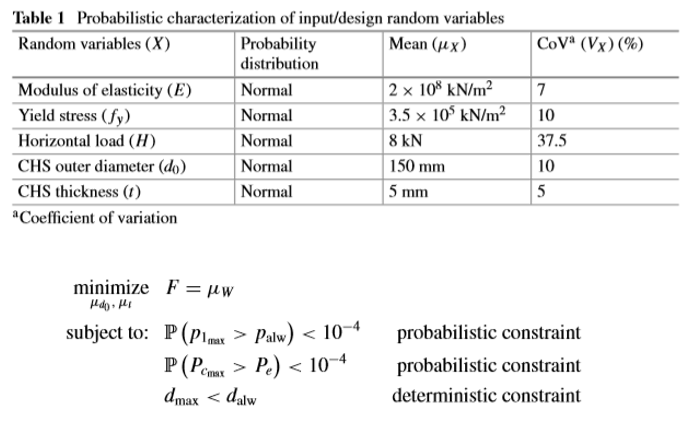# RBDO using PCE

Hi, UQLab!!My major is chemical engineering and I am using data simulation programs. However, data extraction takes a long time because it uses a CFD program that includes formulas such as hydrodynamics.

I am interested in studying RBDO for optimal design of reactor. It was very interesting to see an example of RBDO using MC. However, MC requires too much data compared to PCE, making it difficult to incorporate into my research.
So i think that the best way is PCE.

Is Data Driven RBDO using PCE available in UQLab?
I want to set the failure probability numerically like 0.001 to get the optimum values ​​and optimal design variables of the objective function.

Source : Reliability-Based Design Optimization of a Large-Scale Truss Structure Using Polynomial Chaos Expansion Metamodel, 2020

Hi @Chemicaleng,

Yes you can use PCE for solving RBDO problems with UQLab. Please have a look at the example 04 of the RBDO module (Surrogate-assisted RBDO, Section 4.2 - https://www.uqlab.com/rbdo-surrogate-assisted-rbdo ) for more details how to set up the analysis.
Basically all you need to do is to choose the size of the initial experimental design and set the options of the PCE model. The module will then adaptively build the surrogate model with the aim of accurately representing the limit-state surface. Once this is done, the reliability-based design optimization will be carried out using the built PCE instead of the original model.

I would suggest you also have a look at the possibility of using Kriging instead of PCE.

Cheers,
Moustapha

Thank you for your kind replyI read the Surrogate-assisted RBDO you said.
But what I said is, like Data driven PCE, it’s a way of optimizing only with Data.
However, Surrogate-assisted RBDO seems to be a case of knowing the limit state function of the system in advance, not using only data. However, I thought that the limit state function may not be known in a complex system.

1. Is data driven (Only surrogate) RBDO (not Surrogate-assisted) possible?

2. UQLab ’s example

design variable: d1, d2 (gaussian distribution with only standard deviation)
cost function = d1 + d2
and three limit state function

2- (1) When declaring the distribution of design variable, is there any reason to use only standard deviation without using mean?

2- (2) When using PCE, the code is shown below.
PCEOpts. Degree = 2:10;
PCEOpts.ExpDesign.NSamples = 10;
PCEOpts.ExpDesign.Sampling = ‘LHS’;
Is it correct to see that the above code extracts 10 experimental designs from the range of d1 and d2 initially declared as gaussian using LHS technique and builds a PCE model?
If yes, is it possible to establish a Data driven PCE model if you already have a lot of data?As stated above, it was declared that the optimal solution was derived by adding up to 50 data. If you look at the results in the figure below, you can see that it ends in 9 samples. Then, is it possible that PCE is the optimal solution among the three methods (SVR, PCE, and Kriging)?

1. The figure above is the result of an example.
The cost (Fstar) is almost the same, but the optimal design and constraints at solution are different. In my opinion, if all three methods resulted in an optimal solution, the cost, optimal design and constraints at solution should be similar. How can you tell which method led to the best solution?

Source: Reliability-Based Design Optimization of a Large-Scale Truss Structure Using Polynomial Chaos Expansion Metamodel

It was prepared with reference to the above literature.p 1max : maximum principal axial stress
p alw : allowable axial stress
p cmax : maximum axial compressive force
p e : critical Euler buckling force in compression
d max : maximum value of nodal displacement
d max : allowable deterministic value

As shown in the picture above,
Is it possible to set the probability of failure and find the optimal solution using uqlab?
(only using data, not defining limit state function and cost function)

It seems to me that the method is different from uqlab’s surrogate assisted RBDO.

1. The presence of uncertainty, not a design variable
2. Established a data-driven model without the use of formulas
( ex. limit state function, cost function…)

Hi @Chemicaleng,
In short, yes you can perform RBDO with data-driven PCE in UQLab. I will go through your questions point by point now.

2-(1) We set only the standard deviation because we assume that the mean value is the current value of the design during optimization. Alternatively you can also set a coefficient of variation, then the standard deviation is dependent on the current design at each iteration step.

2-(2) If you already have data, say X and Y and you would like to use them, then you just need to replace
PCEOpts.ExpDesign.NSamples = 10;
PCEOpts.ExpDesign.Sampling = ‘LHS’;
with
PCEOpts.ExpDesign.X = X;
PCEOpts.ExpDesign.Y = Y;

1. Yes indeed, if the analysis converges to the optimal solution, the cost, design and constraints should be very similar. However here we are using surrogates which have different degrees of accuracy, hence the different results. As such I don’t think it is possible to say which one is the best without using a validation set. An alternative would be to use adaptive surrogate model so that the accuracy of the surrogate models are controlled in the vicinity of the limit-state surface.

Cheers,
Moustapha

Hi @Chemicaleng,

Is it possible to set the probability of failure and find the optimal solution using uqlab?
(only using data, not defining limit state function and cost function)

–> Yes you can do that using UQLab’s RBDO as I explained in the previous post.

1. The presence of uncertainty, not a design variable
–> I am not sure I understood your point here. In general, you can use both settings in UQLab, i.e. design variables associated with uncertainty or deterministic design variables. In the later case, you can either set the distribution to “deterministic” or assign a std of 0 to the corresponding design variable.

2. Established a data-driven model without the use of formulas
( ex. limit state function, cost function…)
–> The surrogate-assisted RBDO directly relies on the available surrogate models modules in UQLab. So yes you don’t necessarily need a model. If you only have data you can provide them and a surrogate model will be built a priori before starting the optimization.

Cheers,
Moustapha